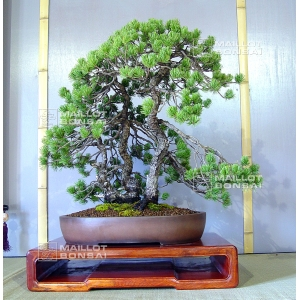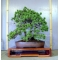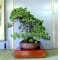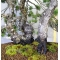The Japanese Bonsai specialist
Direct order Contact Help / Services NewsletterPinus pentaphylla bonsai ref: 13040151

› Outdoor bonsai trees › Bonsai masterpiecesref. : 9382

Product unavailable

Description

Height of the tree: 60 cm.

Width: 58 cm.

Non-enamelled brown pot: 47*38*9 cm.

Table not included.

One of our masterpieces, this pinus pentaphylla or five needle pine is 60-80 years old. Its grand age is reflected in the natural jin. This tree comes from the Japanese nursery of the renowned bonsai master Shiino Kentaro. It was grown from a seedling and has been trained in kabudachi three-trunk style, with short needles and impeccable bark. The winding trunks emerge from a beautiful root base and there are no major wounds or signs of cut branches. This high quality specimen can continue to be refined and worked upon by wire binding its branches. Requires full sun.

#bonsai 5.6 #masterpieces 4.2 #pentaphylla 4.1 #pinus 3.5 #this 3.4 #from 3.4 #branches 2.8 #tree 2.4 #impeccable 2 #reflected 1.9

Formule
(( ROUND((CHAR_LENGTH(b.article_nom)-CHAR_LENGTH(REPLACE(b.article_nom, 'pentaphylla', '')))/LENGTH('pentaphylla')) + ROUND((CHAR_LENGTH(b.article_description)-CHAR_LENGTH(REPLACE(b.article_description, 'pentaphylla', '')))/LENGTH('pentaphylla')) ) * 4.1) + (( ROUND((CHAR_LENGTH(b.article_nom)-CHAR_LENGTH(REPLACE(b.article_nom, 'bonsai', '')))/LENGTH('bonsai')) + ROUND((CHAR_LENGTH(b.article_description)-CHAR_LENGTH(REPLACE(b.article_description, 'bonsai', '')))/LENGTH('bonsai')) ) * 3.6) + (( ROUND((CHAR_LENGTH(b.article_nom)-CHAR_LENGTH(REPLACE(b.article_nom, 'pinus', '')))/LENGTH('pinus')) + ROUND((CHAR_LENGTH(b.article_description)-CHAR_LENGTH(REPLACE(b.article_description, 'pinus', '')))/LENGTH('pinus')) ) * 3.5) + (( ROUND((CHAR_LENGTH(b.article_nom)-CHAR_LENGTH(REPLACE(b.article_nom, 'from', '')))/LENGTH('from')) + ROUND((CHAR_LENGTH(b.article_description)-CHAR_LENGTH(REPLACE(b.article_description, 'from', '')))/LENGTH('from')) ) * 3.4) + (( ROUND((CHAR_LENGTH(b.article_nom)-CHAR_LENGTH(REPLACE(b.article_nom, 'this', '')))/LENGTH('this')) + ROUND((CHAR_LENGTH(b.article_description)-CHAR_LENGTH(REPLACE(b.article_description, 'this', '')))/LENGTH('this')) ) * 3.4) + (( ROUND((CHAR_LENGTH(b.article_nom)-CHAR_LENGTH(REPLACE(b.article_nom, 'branches', '')))/LENGTH('branches')) + ROUND((CHAR_LENGTH(b.article_description)-CHAR_LENGTH(REPLACE(b.article_description, 'branches', '')))/LENGTH('branches')) ) * 2.8) + (( ROUND((CHAR_LENGTH(b.article_nom)-CHAR_LENGTH(REPLACE(b.article_nom, 'tree', '')))/LENGTH('tree')) + ROUND((CHAR_LENGTH(b.article_description)-CHAR_LENGTH(REPLACE(b.article_description, 'tree', '')))/LENGTH('tree')) ) * 2.4) + (( ROUND((CHAR_LENGTH(b.article_nom)-CHAR_LENGTH(REPLACE(b.article_nom, 'masterpieces', '')))/LENGTH('masterpieces')) + ROUND((CHAR_LENGTH(b.article_description)-CHAR_LENGTH(REPLACE(b.article_description, 'masterpieces', '')))/LENGTH('masterpieces')) ) * 2.2) + (( ROUND((CHAR_LENGTH(b.article_nom)-CHAR_LENGTH(REPLACE(b.article_nom, 'impeccable', '')))/LENGTH('impeccable')) + ROUND((CHAR_LENGTH(b.article_description)-CHAR_LENGTH(REPLACE(b.article_description, 'impeccable', '')))/LENGTH('impeccable')) ) * 2) + (( ROUND((CHAR_LENGTH(b.article_nom)-CHAR_LENGTH(REPLACE(b.article_nom, 'beautiful', '')))/LENGTH('beautiful')) + ROUND((CHAR_LENGTH(b.article_description)-CHAR_LENGTH(REPLACE(b.article_description, 'beautiful', '')))/LENGTH('beautiful')) ) * 1.9)

Secure paymentDelivery

Our logistic partners :04 74 55 23 48
Pépinière MAILLOT-BONSAÏ
Le Bois Frazy
01990 RELEVANT - FRANCE
on appointment